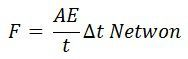# Piezo-Electric Transducer

Definition: The Piezoelectric transducer is an electroacoustic transducer use for conversion of pressure or mechanical stress into an alternating electrical force. It is used for measuring the physical quantity like force, pressure, stress, etc., which is directly not possible to measure.

The piezo transducer converts the physical quantity into an electrical voltage which is easily measured by analogue and digital meter.

The piezoelectric transducer uses the piezoelectric material which has a special property, i.e. the material induces voltage when the pressure or stress applied to it. The material which shows such property is known as the electro-resistive element.

The word piezoelectric means the electricity produces by the pressure. The Quartz is the examples of the natural piezoelectric crystals, whereas the Rochelle salts, ammonium dehydration, phosphate, lithium sulphate, dipotassium tartrate are the examples of the man made crystals. The ceramic material is also used for piezoelectric transducer.

The ceramic material does not have the piezoelectric property. The property is developed on it by special polarizing treatment. The ceramic material has several advantages. It is available in different shapes and sizes. The material has the capability of working at low voltages, and also it can operate at the temperature more than 3000ºC

## Piezoelectric Effect

The EMF develops because of the displacement of the charges. The effect is changeable, i.e. if the varying potential applies to a piezoelectric transducer, it will change the dimension of the material or deform it. This effect is known as the piezoelectric effect.

The pressure is applied to the crystals with the help of the force summing devices for examples the stress is applied through mechanical pressure gauges and pressure sensors, etc. The deformation induces the EMF which determines the value of applied pressure.

### Theory of Piezo-Electric Transducer

A piezoelectric crystal is shown in the figure below.The polarity of the charge depends on the direction of the applies forces.Where, d – charge sensitivity of the crystals
F – applied force in Newton

The force changes the thickness of the crystals.Where A – area of crystals in meter square
t – the thickness of crystals in meter
E –  Young’s modulus N/m2

where ω – width of crystals in meter
l – the length of crystals in meter

On substituting the value of force in the equation of charge, we getThe output voltage is obtained because of the electrode charges.The g is the voltage sensitivity of the crystals.Where E0 – electric field strength, V/m

The voltage sensitivity of the crystals is expressed by the ratio of the electric field intensity and pressure.

When the mechanical deformation occurs in the crystals, it generates charges. And this charge develops the voltages across the electrodes.

The Piezoelectric crystal is direction sensitive. The polarity of the voltage depends on the direction of the force which is either tensile or compressive. The magnitude and the polarity of the charges depend on the magnitude and the direction of the applied force.

### Modes of Operation of Piezo-Electric Crystal

The Piezoelectric crystals are used in many modes likes, thickness shear, face shear, thickness expansion, Transverse expansion, etc. The figure of the fear shear is shown in the figure below.### Properties of Piezo Electric-Crystal

The following are the properties of the Piezoelectric Crystals.

1. The piezoelectric material has high stability.
2. It is available in various shapes and sizes.
3. The piezoelectric material has output insensitive to temperature and humidity.

### Uses of Piezoelectric Crystal

The following are the uses of the Piezoelectric transducers.

1. The piezoelectric material has high stability and hence it is used for stabilizing the electronic oscillator.
2. The ultrasonic generators use the piezoelectric material. This generator is used in SONAR for underwater detection and in industrials apparatus for cleaning.
3. It is used in microphones and speakers for converting the electric signal into sound.
4. The piezoelectric material is used in electric lighter.

The transducer has low output, and hence external circuit is associated with it.

### 7 thoughts on “Piezo-Electric Transducer”

1. It gave me a complete idea about the topic which I was searching for. It also provided me with a simple and easy definition

2. Does the electric voltage corresponds to the amount of mechanical force. In other words can we quantify the pressure from electric voltage and how?

3. My another question is that the how faithfully does the electric voltage wave generated on an oscilloscope represents the pressure wave input from a piezoelectric transducer

4. sir please reply me about how to enhance the output of the piezoelectric transducer in order to use it for a high voltage purpose?